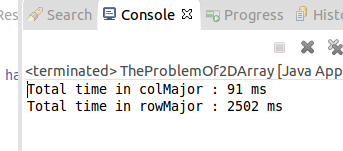{{announcement.body}}
{{announcement.title}}

# Multidimensional Array Traversal in Java

DZone 's Guide to

# Multidimensional Array Traversal in Java

### Learn more about how to handle arrays in Java, and some of the misconceptions surrounding the objects.

· Java Zone ·
Free Resource

Comment (4)

Save
{{ articles.views | formatCount}} Views

### Multidimentional Arrays

An array is a container object in Java that holds a fixed number of values of a single type. The length of an array is established when the array is created. After creation, its length is fixed. e.g. an array of size 10 is defined below:

``````
public class ArrayDemo {
private int arraySize=10;
public int[] arrayOfIntegers = new int[arraySize];
}``````

The above code is an example of single dimentional array. In other words the array grows only in one direction. Many a times we need arrays that grow in more than one dimention. Such arrays are called multi-dimentional arrays. For simplicity, let's talk about a 2-D array. 2-D arrays are very useful when we need a matrix or x-y plots/graphs. Below is an example of a square 2-D array.

``````public class TheProblemOf2DArray {

private static final int ARR_SIZE=10;
public static void main(String[] args) {
int arr[][]=new int[ARR_SIZE][ARR_SIZE];
}
}``````

To imagine, a 2-D array looks like a matrix of x and y co-ordinates.However there is a little surprise for Java developers. Java does not actually have 2 arrays.

In a true array, all the elements of the array occupy a continuous block of memory, but that's not true in case of 2D arrays in Java. All the elements in a 1-D array in java occupies adjacent memory locations, hence it is indeed a true array.

In Java, when we define:

``````int singleElement  // this means an int variable
int[] singleDArray // this means an array of int variables (from 1)
int[][] twoDArray // this means an array of arrays of int variables (from 2)``````

It means that in the above example, twoDArray is a reference to an array, whose each element is a reference to another array of int elements.

This image explains the concept very nicely.

Since a 2-D array is scattered in memory, it has some impacts on performance. To analyze such differences, I have written a simple Java program which depicts the importance of traversal order.

``````package arrayTraverse;
/**
* The problem of 2 D array.
*
* Here we are initializing a 2 D array of arbitrary size. (For simplycity we have used a square 2 D array)
* We are going to iterate the same array in two ways and analyse the outcome
*
* Their is huge performance difference in both type of iterations
*
* @author mohit
*
*/
public class TheProblemOf2DArray {
// Array size: bigger the size, clearer is the difference in performance
private static final int ARR_SIZE=9999;
public static void main(String[] args) {
//new array
int arr[][]=new int[ARR_SIZE][ARR_SIZE];
long currTime=System.currentTimeMillis();
colMajor(arr);
System.out.println("Total time in colMajor : "+(System.currentTimeMillis()-currTime)+" ms");

// new array, exactly similar to arr
int arr1[][]=new int[ARR_SIZE][ARR_SIZE];
currTime=System.currentTimeMillis();
rowMajor(arr1); // this is the only difference in above
System.out.println("Total time in col : "+(System.currentTimeMillis()-currTime) +" ms");
}
/**
* The code below traverses the matrix in column-major order,
* i.e. it completely sweeps one column before going to the next.
*
*/
private static void colMajor(int arr[][]) {
for(int i=0;i<ARR_SIZE;i++){
for (int j=0;j<ARR_SIZE;j++){
/*See this, we are traversing j first and then i*/
arr[i][j]=i+j;
}
}

}
/**
* If we switch the inner and outer loops,
* the program will traverse the matrix in row-major order,
* i.e. it will sweep each row before going to a new one,
* which means it accesses a different column (and hence a different page) every time it accesses the array.
* This slight change to the code caused the program to take more time to complete.
*/
private static void rowMajor(int arr[][]) {
for(int i=0;i<ARR_SIZE;i++){
for (int j=0;j<ARR_SIZE;j++){
/*See this , we are traversing j first and then i, but to access the
* element, more effort is involved now as they are farther located
*/
arr[j][i]=i+j;
}
}
}
}``````

Below is a sample output:The above example is repeatable and it consistently gives similar output, however the time difference may vary.

Topics:
java, memory access pattern, performanace, traversal

Comment (4)

Save
{{ articles.views | formatCount}} Views

Opinions expressed by DZone contributors are their own.